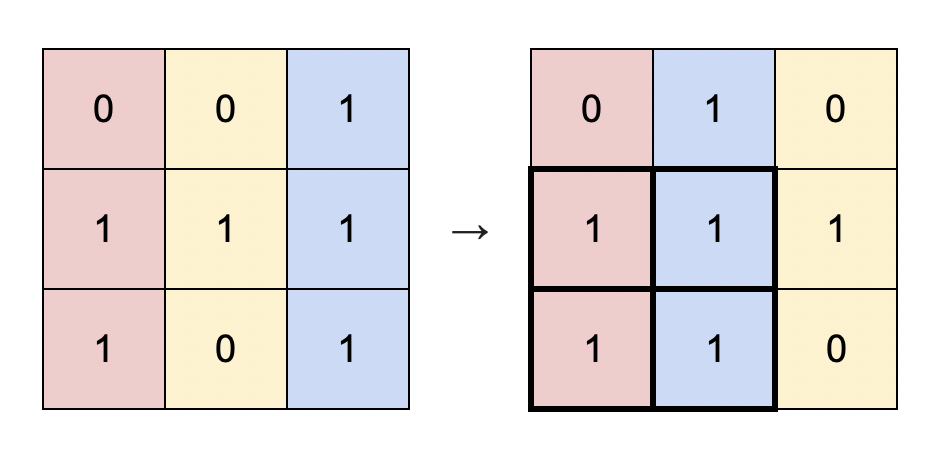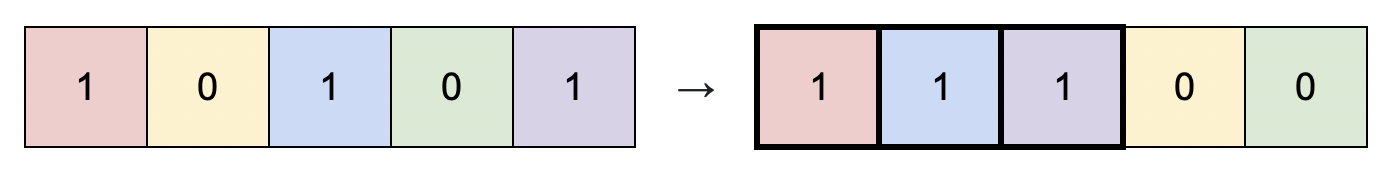1727. Largest Submatrix With Rearrangements

You are given a binary matrix `matrix` of size `m x n`, and you are allowed to rearrange the columns of the `matrix` in any order.

Return the area of the largest submatrix within `matrix` where every element of the submatrix is `1` after reordering the columns optimally.

Example 1:```Input: matrix = [[0,0,1],[1,1,1],[1,0,1]]
Output: 4
Explanation: You can rearrange the columns as shown above.
The largest submatrix of 1s, in bold, has an area of 4.
```

Example 2:```Input: matrix = [[1,0,1,0,1]]
Output: 3
Explanation: You can rearrange the columns as shown above.
The largest submatrix of 1s, in bold, has an area of 3.
```

Example 3:

```Input: matrix = [[1,1,0],[1,0,1]]
Output: 2
Explanation: Notice that you must rearrange entire columns, and there is no way to make a submatrix of 1s larger than an area of 2.```

Example 4:

```Input: matrix = [[0,0],[0,0]]
Output: 0
Explanation: As there are no 1s, no submatrix of 1s can be formed and the area is 0.```

Constraints:

• `m == matrix.length`
• `n == matrix[i].length`
• `1 <= m * n <= 105`
• `matrix[i][j]` is `0` or `1`.

1727. Largest Submatrix With Rearrangements
``````struct Solution;

impl Solution {
fn largest_submatrix(matrix: Vec<Vec<i32>>) -> i32 {
let n = matrix.len();
let m = matrix.len();
let mut rows = vec![vec![0; m]; n];
for i in 0..n {
for j in 0..m {
if matrix[i][j] == 1 {
rows[i][j] = if i > 0 { rows[i - 1][j] } else { 0 } + 1;
}
}
}
let mut res = 0;
for i in 0..n {
res = res.max(Self::rearrange(&mut rows[i]));
}
res
}

fn rearrange(row: &mut Vec<i32>) -> i32 {
row.sort_unstable();
let m = row.len();
let mut max = 0;
for i in 0..m {
max = max.max(row[i] * (m - i) as i32);
}
max
}
}

#[test]
fn test() {
let matrix = vec_vec_i32![[0, 0, 1], [1, 1, 1], [1, 0, 1]];
let res = 4;
assert_eq!(Solution::largest_submatrix(matrix), res);
let matrix = vec_vec_i32![[1, 0, 1, 0, 1]];
let res = 3;
assert_eq!(Solution::largest_submatrix(matrix), res);
let matrix = vec_vec_i32![[1, 1, 0], [1, 0, 1]];
let res = 2;
assert_eq!(Solution::largest_submatrix(matrix), res);
let matrix = vec_vec_i32![[0, 0], [0, 0]];
let res = 0;
assert_eq!(Solution::largest_submatrix(matrix), res);
}
``````